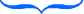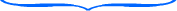# Scientific Notation of Large Numbers

Sometimes we are faced with very large numbers. For example, take a look at the mass of the Earth.

5,972,000,000,000,000,000,000,000kg

When astronomers are doing calculations with such large numbers, it can become quite difficult to keep track of all the zeros.

There is another way to write such large numbers that makes working with them much easier. This method is known as scientific notation. It is a special notation that helps us work with scientific numbers.

To use scientific notation, we need to know about powers of ten:

100 = 1

101 = 10

102 = 100

103 = 1,000

104 = 10,000

When we have large numbers, we can rewrite them as powers of ten.

Example:30,000 = 3 x 10,000

Next, we can rewrite this using an exponent.3 x 10,000 = 3 x 104

You can see that this number is already simpler because there a much less zeros to work with.

This number,3 x 104, is an example of a number written in scientific notation.

To write a number in scientific notation, the first part of the product should be between 1 and 10 and then second part should be a power of ten.

# less than 10 x power of ten or # less than ten x 10?

Let's take a look at a few more examples:

1.)25,000,000

Step 1: Move the decimal between the first two numbers.

2.5,000,000

Step 2: Count how many times the decimal had to move.

2.5000000.The decimal was at the end of the whole number. We had to move seven times to be in between the 2 and 5. So the exponent will be 7.

The decimal was at the end of the whole number. We had to move seven times to be in between the 2 and 5. So the exponent will be 7.

Step 3:Write the answer in scientific notation.

2.5 x 107

2.)43,900,000,000,000

Step 1:Get the decimal between the 4 and 3.

4.3,900,000,000,000

Step 2:Count the number of moves from the end to between the 4 and 3.

4.3,900,000,000,000.We would have to move 13 times.4.39 x 1013

We can also convert back to standard form when given a number in scientific notation.

Examples:

1.)4.7 x 103

Step 1:Take note of your exponent. We have a 3.

Step 2:Move the decimal to the right three times because the exponent was a three.

4,700 This is the number in standard form.

**Notice that the exponent is the number of moves, not the number of zeros!!!**

2.)7.05 x 105

Step 1:Notice that the exponent is a 5.

Step 2:Now move the decimal 5 times to the right.

705,000 This is our number in standard form.

Let's Review: Scientific notation is a great way to rewrite numbers with lots of zeros so that they are easier to work with. Numbers written in scientific notation have two parts. The first part is a number less than ten, but greater than 1. Then this is multiplied times a power of ten. The exponent tells us how many times the decimal has moved, not the number of zeros in the number!